# Multiple Regression Analysis: A prediction framework

## Objectives

It is well accepted that functional connectivity and structural brain connectivity are interlinked and they are both affected in neurological diseases. It has been also shown that strong structural connections are typically associated with strong functional connections between regions. Given a set of ROIs and the associated connections between each pair of ROIs we would like to construct a systematic framework to characterise the link between functional and structural connectivity and answer the following questions.

• Can we predict changes in functional connectivity from changes in structural connectivity? Then we could potentially estimate the functional dysfunction once we have identified structural abnormalities.

• Can we identify reliably the structural connections that affect a specific functional connection and isolate the effect that one connection has on another? Then we can invalidate causal alternatives and help researchers in choosing between competing theories.

• Can we answer these questions with a rigorous statistical framework? Then we can generalise our conclusions from a sample set of subjects to the general population.

## Multiple Regression Analysis

We suggest a prediction framework to study the link between structural brain connectivity and functional brain connectivity. A key advantage of this approach is that it describes influences of brain structure on function within a rigorous statistical framework. This data driven approach does not depend on a-priori hypothesis and thus, can facilitate the development of robust connectivity-based biomarkers. In this framework, each functional connection,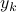is a dependent variable, which is described as a linear combination of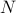structural connections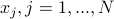, also called independent variables or prediction variables. The goal is to estimate the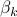parameters that provide the best least square fit over all subjects,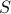, of the linear equation: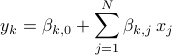Multiple regression is a statistical method for studying the relationship between a single dependent variable and several independent variables. It is used both for prediction and causal analysis:

• In a prediction the goal is to construct a mathematical formula that estimates the dependent variable based on the observed independent variables.

• In a causal analysis the independent variables are considered as causes of the dependent variable. The aim of the study is to detect whether a particular independent variable affects the dependent variable and to estimate the magnitude of this effect, if any.

Multiple regression is extremely popular in several sciences including social sciences, psychology, biology and clinical science. This is because:

• In prediction studies, multiple regression makes it possible to combine many variables to produce optimal predictions of the dependent variable.

• In causal analysis, it separates the effects of independent variables on the dependent variable so that you can examine the unique contribution of each variable.

Although, multiple regression is based on a linear equation, it can describe complex relationships between structure and function. In the absence of any prior knowledge, a linear equation is the simplest way to describe a relationship between structure and function and still get reasonably accurate predictions. Note that in multiple regression the dependent variables can also take non-linear forms. Here, we are not going to explore this further. Also note that multiple regression analysis can analyse any dataset tailored for ANOVA, whereas the opposite is not always true. It yields identical statistical tests to those provided by ANOVA yet it offers additional measures of effect size and it often permits more straightforward interpretation.

## Sparse Multiple Regression Analysis

Evidence that the relationship between functional and structural connectivity is sparse come from recent work in traumatic brain injury that shows that the integrity of a structural connection, between the right anterior insula and the pre-supplamentary motor area, predicts function within the default mode network, exclusively (Bonnelle et al., PNAS, 2012).

A sparse representation over least square multiple regression it offers two major advantages that are very useful in modelling brain connectivity:

• It improves prediction by setting some coefficients to zero. This results in removing noisy and irrelevant variables and thus reducing the total variance.

• It allows the selection of the most relevant variables and thus it links each functional connection with a subset of structural connection in a data driven way.

We use the Least Absolute Shrinkage and Selective Operator (LASSO) to impose an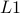penalty on the multiple regression that controls its sparsity. LASSO can be implemented with computationally efficient ways and it controls sparsity with just one parameter,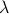. Whengets high values most of the coefficients are set to zero and the corresponding structural connections are eliminated. The remaining structural connections are those strongly linked with the functional connection: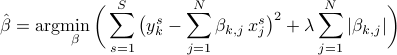The correct predictors are identified with high probability even when the number of variables is higher than the number of observation under the assumption that functional brain connectivity can have a true sparse representation based on structural connectivity.